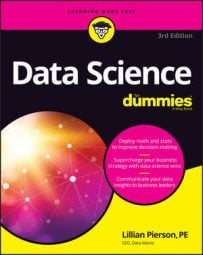##### Data Science For DummiesIf statistics has been described as the science of deriving insights from data, then what’s the difference between a statistician and a data scientist? Good question! While many tasks in data science require a fair bit of statistical know how, the scope and breadth of a data scientist’s knowledge and skill base is distinct from those of a statistician. The core distinctions are outlined below.
• Subject matter expertise: One of the core features of data scientists is that they offer a sophisticated degree of expertise in the area to which they apply their analytical methods. Data scientists need this so that they’re able to truly understand the implications and applications of the data insights they generate. A data scientist should have enough subject matter expertise to be able to identify the significance of their findings and independently decide how to proceed in the analysis.

In contrast, statisticians usually have an incredibly deep knowledge of statistics, but very little expertise in the subject matters to which they apply statistical methods. Most of the time, statisticians are required to consult with external subject matter experts to truly get a firm grasp on the significance of their findings, and to be able to decide the best way to move forward in an analysis.

• Mathematical and machine learning approaches: Statisticians rely mostly on statistical methods and processes when deriving insights from data. In contrast, data scientists are required to pull from a wide variety of techniques to derive data insights. These include statistical methods, but also include approaches that are not based in statistics — like those found in mathematics, clustering, classification, and non-statistical machine learning approaches.

## Seeing the importance of statistical know-how

You don't need to go out and get a degree in statistics to practice data science, but you should at least get familiar with some of the more fundamental methods that are used in statistical data analysis. These include:
• Linear regression: Linear regression is useful for modeling the relationships between a dependent variable and one or several independent variables. The purpose of linear regression is to discover (and quantify the strength of) important correlations between dependent and independent variables.

• Time-series analysis: Time series analysis involves analyzing a collection of data on attribute values over time, in order to predict future instances of the measure based on the past observational data.

• Monte Carlo simulations: The Monte Carlo method is a simulation technique you can use to test hypotheses, to generate parameter estimates, to predict scenario outcomes, and to validate models. The method is powerful because it can be used to very quickly simulate anywhere from 1 to 10,000 (or more) simulation samples for any processes you are trying to evaluate.

• Statistics for spatial data: One fundamental and important property of spatial data is that it’s not random. It’s spatially dependent and autocorrelated. When modeling spatial data, avoid statistical methods that assume your data is random. Kriging and krige are two statistical methods that you can use to model spatial data. These methods enable you to produce predictive surfaces for entire study areas based on sets of known points in geographic space.

## Working with clustering, classification, and machine learning methods

Machine learning is the application of computational algorithms to learn from (or deduce patterns in) raw datasets. Clustering is a particular type of machine learning —unsupervised machine learning, to be precise, meaning that the algorithms must learn from unlabeled data, and as such, they must use inferential methods to discover correlations.

Classification, on the other hand, is called supervised machine learning, meaning that the algorithms learn from labeled data. The following descriptions introduce some of the more basic clustering and classification approaches:

• k-means clustering: You generally deploy k-means algorithms to subdivide data points of a dataset into clusters based on nearest mean values. To determine the optimal division of your data points into clusters, such that the distance between points in each cluster is minimized, you can use k-means clustering.

• Nearest neighbor algorithms: The purpose of a nearest neighbor analysis is to search for and locate either a nearest point in space or a nearest numerical value, depending on the attribute you use for the basis of comparison.

• Kernel density estimation: An alternative way to identify clusters in your data is to use a density smoothing function. Kernel density estimation (KDE) works by placing a kernel a weighting function that is useful for quantifying density — on each data point in the data set, and then summing the kernels to generate a kernel density estimate for the overall region.

## Keeping mathematical methods in the mix

Lots gets said about the value of statistics in the practice of data science, but applied mathematical methods are seldom mentioned. To be frank, mathematics is the basis of all quantitative analyses. Its importance should not be understated. The two following mathematical methods are particularly useful in data science.
• Multi-criteria decision making (MCDM): MCDM is a mathematical decision modeling approach that you can use when you have several criteria or alternatives that you must simultaneously evaluate when making a decision.

• Markov chains: A Markov chain is a mathematical method that chains together a series of randomly generated variables that represent the present state in order to model how changes in present state variables affect future states.